Stiffened equation of state

The Stiffened equation of state is a simplified form of the Grüneisen equation of state . When considering water under very high pressures (typical applications are underwater explosions, extracorporeal shock wave lithotripsy, and sonoluminescence) the stiffened equation of state is often used: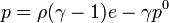$p=\rho(\gamma-1)e-\gamma p^0$

where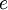$e$ is the internal energy per unit mass, given by (Eq. 15 in ):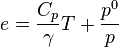$e = \frac{C_p}{\gamma}T + \frac{p^0}{p}$

where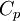$C_p$ is the heat capacity at constant pressure.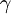$\gamma$ is an empirically determined constant typically taken to be about 6.1, and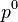$p^0$ is another constant, representing the molecular attraction between water molecules. The magnitude of the later correction is about 2 gigapascals (20,000 atmospheres).

The equation is stated in this form because the speed of sound in water is given by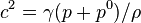$c^2=\gamma(p+p^0)/\rho$.

Thus water behaves as though it is an ideal gas that is already under about 20,000 atmospheres (2 GPa) pressure, and explains why water is commonly assumed to be incompressible: when the external pressure changes from 1 atmosphere to 2 atmospheres (100 kPa to 200 kPa), the water behaves as an ideal gas would when changing from 20,001 to 20,002 atmospheres (2000.1 MPa to 2000.2 MPa).

This equation mispredicts the heat capacity of water but few simple alternatives are available for severely nonisentropic processes such as strong shocks.